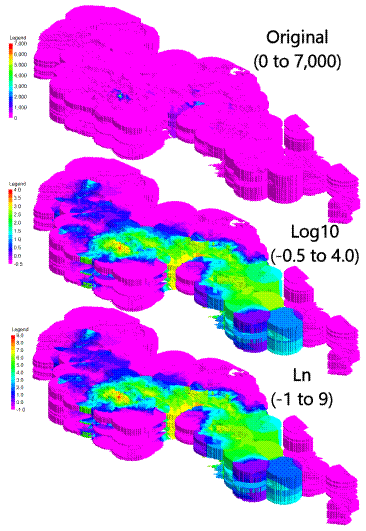Math Options

Model + Constant:  Add a specified constant to every model node.

Model + Model:          Add the corresponding node values from another model.  Example: Add two thickness grid models to output total thickness values.

Model – Constant:  Subtract a specified constant from every node.

Constant – Model:  Subtract every node value from a constant value.

Model – Model:  Subtract the corresponding node values from another model.  Example: Subtract a lower surface grid from an upper grid to output thickness values.

Model x Constant:  Multiply every node by a specified constant.

Model x Model:  Multiply every node by the corresponding node value within another model.  Example: Multiply a model with a Boolean model (containing 0's and 1's) to zero-out areas where defined criteria are not met.

Model / Constant:  Divide every node by a specified constant.  If the Constant value equals zero, C will equal zero.

Constant / Model:  Divide a constant by every node value.

Model / Model:  Divide every node by the corresponding node value within another model.  If the B value equals zero, C will equal zero (i.e., no divide-by-zero error).  Example: Divide an ore thickness grid by an overburden thickness grid to create a stripping ratio model.

Absolute:  Convert all node values to their absolute values.

Average:  Compute the average for nodes within two models.  Example: Compute average hydrothermal temperatures between two different dates.

Difference:  Compute the difference between two models by computing the absolute value of subtracted nodes.

Highest:  If A > B then Result = A else Result = B.

Lowest:  If A < B then Result = A else Result = B.

Boolean AND:  If A = 1 & B = 1 then Result = 1 else Result = 0.  Only applies to Boolean models.

Boolean OR:  If A = 1 or B = 1 then Result = 1 else Result = 0.  Only applies to Boolean models.

Boolean XOR:  If A <> B then Result = 1 else Result = 0.  Only applies to Boolean models.

Log10: Returns the log base 10 of the node values (see example below).

Ln: Returns the natural logarithm of the node values (see example below).